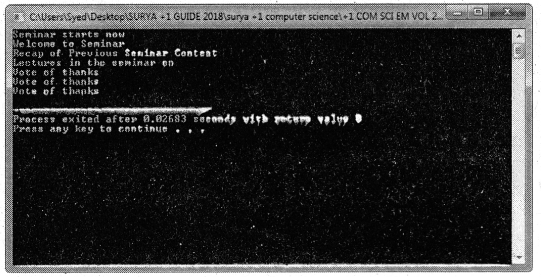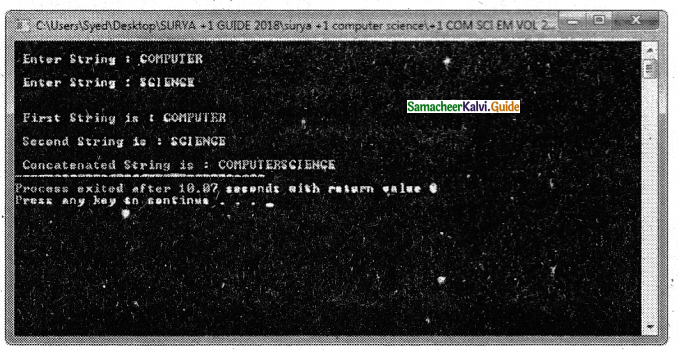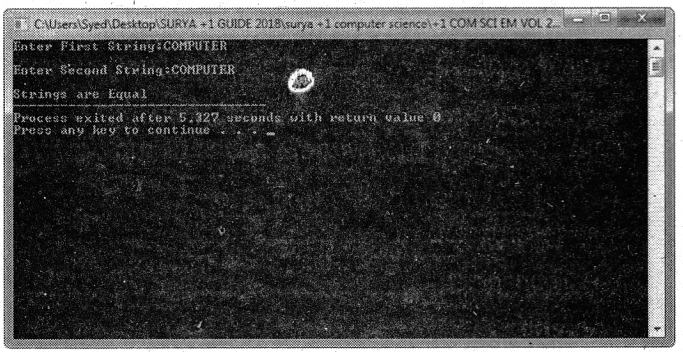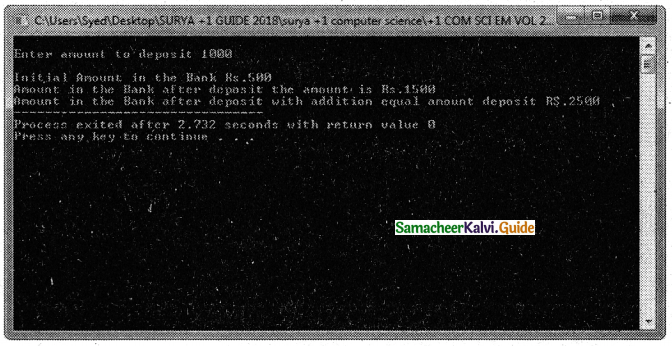Tamilnadu State Board New Syllabus Samacheer Kalvi 11th Computer Science Guide Pdf Chapter 15 Polymorphism Text Book Back Questions and Answers, Notes.

## Tamilnadu Samacheer Kalvi 11th Computer Science Solutions Chapter 15 Polymorphism

### 11th Computer Science Guide Polymorphism Text Book Questions and AnswersPart I

Question 1.
Which of the following refers to a function having more than one distinct meaning?

Question 2.
Which of the following reduces the number of comparisons in a program?Question 3.
void dispchar(char ch=’$’,int size=10) ‘ { for(int i=1;i<=size;i++) cout<<ch; } How will you invoke the function dispchar() the following input? To print$ for 10 times
a) dispchar();
b) dispchar(ch,size);
c) dispchar($,10); d) dispcharC$’, 10 times);
a) dispchar();

Question 4.
Which of the following is not true with respect to function overloading?
a) The overloaded functions must differ in their signature.
d) Destructor function cannot be overloaded.

Question 5.
a) void fun (int x);
void fun (char ch);.
b) void fun (int x);
void fun (int y);
c) void fun (double d);
void fun (char ch);
d) void fun (double d);
void fun (int y);
b) void fun (int x);
void fun (int y);Question 6.
Which of the following function(s) combination cannot be considered as overloaded function(s) in the given snippet?
void print(char A,int B); // F1
void printprint(int A, float B); // F2
void Print(int P=10); // F3
void print(); // F4
a) F1,F2,F3,F4
b) F1,F2,F3
c) F1,F2,F4
d) F1,F3,F4
b) F1,F2,F3

Question 7.
Which of the fallowing operator is by default overloaded by the compiler?
a) *
b) +
c) +=
d) ==
c) +=

Based on the following program answer the questions (8) to (10)
#include<iostream>
using namespace std;
class Point
{
private:,
int x, y;
public:
Point(int x1,int y1)
{
x=x1;
y=y1;
}
void operator+(Point &pt3);
void show()
{
cout << “x = ” << x << “, y = “<<y;
}
};
void Point: :operator+(Point &pt3)
{
x += pt3.x;
y += pt3.y;
}
int main()
{
Point pt1(3,2),pt2(5,4);
pt1+pt2;
pt1.show();
return 0;
}Question 8.
Which of the following operator is overloaded?
a) +
b) operator
c) ::
d) =
a) +

Question 9.
a) pt1+pt2;
b) Point pt1(3,2),pt2(5,4);
c) pt1.show();
d) return 0;
a) pt1+pt2;

Question 10.
What is the output for the above program?
a) x=8, y=6
b) x=14, y=14
c) x=8, y=6
d) x=5, y=9
a) x=8, y=6

Part – II

Question 1.
The ability of the function to process the message or data in more than one form is called function overloading. In other words, function overloading means two or more functions in the same scope share the same name but their parameters are different.

Question 2.
List the operators that cannot be overloaded.
The following operators can not be overloaded:

• scope operator::
• sizeof
• member selector.
• member pointer selector *
• ternary operator ?:Question 3.
{
int x;
public:
};

Write an outline definition for the constructor.
OUTLINE CONSTRUCTOR DEFINITION
{
x = a;
cout<<“\nParameterized constructor”;
}

Question 4.

Question 5.

• Function overloading is not only implementing polymorphism but also reduces the number of comparisons in a program and makes the program to execute faster.
• It also helps the programmer by reducing the number of function names to be remembered.
• Program complexity is reduced.Part – III

Question 1.

1. The overloaded function must differ in the number of its arguments or data types.
3. The default arguments of overloaded functions are not considered as part of the parameter list in function overloading.

Question 2.
How does a compiler decide as to which function should be invoked when there are many functions? Give an example.
The number and types of a function’s parameters are called the function’s signature. When we call an overloaded function, the compiler determines the most appropriate definition to use, by comparing the argument types we have used to call the function with the parameter types specified in the definitions. The process of selecting the most appropriate overloaded function or operator is called overload resolution.

Example:
float area (float half, float base, float height);
float area (float length , float breadth);
According to the input passed, the respective function is called.

For example:
x= area(5); // calls area() with one input.
x= area(5,6); // calls area() with two input.
x= area(0.5,5,6);// calls area() with three input.

Question 3.
Example:
The following operators can be overloaded +,++,-,—,+=,-=,*.<,>, etc.

Question 4.
Function overloading can be applied for constructors, as constructors are special functions of classes. A class can have more than one constructor with a different signature. Constructor overloading provides the flexibility in creating multiple types of objects for a class.

1. Memory is allocated for the objects.
2. Initialisation for the objects.Question 5.
class sale
{
int cost, discount;
public:
sale(sale &);
};
Write a non-inline definition for the constructor specified;
Non-inline definition for constructor:
sale :: sale(sale &s)
{
cost = s.cost;
discount = s.discount;
}

Explain In Detail

Question 1.

1. The precedence and associativity of an operator cannot be changed.
2. No new operators can be created, only existing operators can be overloaded.
3. Cannot redefine the meaning of an operator’s procedure. You cannot change how integers are added. Only additional functions can be to an operator.
4. Overloaded operators cannot have default arguments.
5. When binary operators are overloaded, the left-hand object must be an object of the relevant class.

Question 2.
Answer the question (i) to (v) after going through the following class.
classBook
{
int BookCode ;
char Bookname;
float fees;
public:
Book() //Function 1
{
fees =1000;
BookCode=1;
strcpy (Bookname,”C++”);
}
void display(float C) //Function 2
{
cout< < BookCode < < “:”< < Boo kname<<“:”<<fees<<endl;
}
~Book() //Function 3
{
cout<<“End of Book
Object”<<endl;
}
Book (int BC,char S[ ],float F); //
Function 4
};

i) In the above program, what are Function 1 and Function 4 combined together referred to?
ii) Which concept is illustrated by Function3? When is this function called/ invoked?
iii) What is the use of Function3?
iv) Write the statements in main to invoke function1 and function2
v) Write the definition for Function4
i) Constructor
ii) Destructor. It will be executed automatically when object goes out of scope.
iii) To remove the memory space of the object allocated at the time of creation.
iv) a) Book b; // object b automatically call the constructor function Book(); (Function 1)
b) display(4.5); // Invokes the display function by passing 4.5. (Function 2)
v) Definition of Function 4:
Book (int BC,char S[ ],float F)
{
fees=F;
BookCode=BC;
strcpy (Bookname,S);
}Question 3.
Write the output of the following program.
#include<iostream>
using namespace std;
class Seminar
{
int Time;
public:
Seminar()
{
Time=30;cout<<“Seminar starts now”<<endl;
}
void Lecture()
{
cout<<“Lectures in the seminar on”<<endl;
}
Seminar(int Duration)
{
Time=Duration;cout <<“Welcome to Seminar “<<endl;
}
Seminar(Seminar &D)
{
Time=D.Time;cout<<“Recap of Previous Seminar Content “<<endl;
}
~Semina r()
{
cout<<“Vote of thanks”<<endl;
}
};
int main()
{
Seminar s1,s2(2),s3(s2);
s1.LectureQ;
return 0;
}
Output:Question 4.
Debug the following program.
#include<iostream>
using namespace std;
class String
{
public:
charstr;
public:
void accept_string
{
cout<<“\n Enter String :
cin>>str;
}
display_string()
{
cout<<str;
}
String operator *(String x) //Concatenating String
{
String s;
strcat(str,str);
strcpy(s.str,str);
gotos;
}
}
int main()
{
String str1, str2, str3;
str1.accept_string();
str2 .accept_string();
Cout<<“\n\n First String is : “;
str1.display_string();
cout<<“\n\n Second String is : “;
str2.display_string();
str3=str1+str2;
cout<<“\n\n Concatenated String is :”;
str3.display_string();
return 0;
}

Correct Program :

using namespace std;
#include<iostream>
#include<string.h>
class String
{
public:
char str;
public:
void accept_string ()
{
cout<<“\n Enter String :”;
cin>>str;
}
void display__string()
{
cout<<str;
}
String operator+(String x)//
Concatenating String
{
Strings;
strcat(str,x.str);
strcpy(s.str,str);
return(s);
}
};
int main()
{
String str1, str2, str3;
str1.accept_string();
str2 .accept_stri ng();
Cout<<“\n\n First String is : “;
str1.display_string();
cout<<“\n\n Second String is : “;
str2.display_string();
str3=str1+str2;
cout<<“\n\n Concatenated String is :”;
str3.display_string();
return 0;
}

Output:Question 5.
Answer the questions based on the following program.
#include<iostream>
#include<string.h>
using namespace std;
class comp
{
public:
chars;
void getstring(char str)
{
strcpy(s,str);
}
void operator==(comp);
};
void comp::operator==(comp ob)
{
if(strcmp(s,ob.s)==0)
cout<<“\nStrings are Equal”;
else
cout<<“\nStrings are not Equal”;
}
int main()
{
comp ob, ob1;
char stringl, string2;
cout<<“Enter First String:”;
cin>>string1;
ob.getstring(stringl);
cout<<“\nEnter Second String:”;
cin>>string2;
ob1.getstring(string2);
ob==ob1;
return 0; ‘
}

i) Mention the objects which will have the scope till the end of the program.
ii) Name the object which gets destroyed in between the program.
iii) Name the operator which is overloaded and write the statement that invokes it.
iv) Write out the prototype of the overloaded member function
v) What types of operands are used for the overloaded operator?
vi) Which constructor will get executed? Write the output of the program
i) Objects ob and obi in the main( )will have the scope till the end of the program.
ii) Object ob in the operator== function will be destroyed when exit from it.
iii) The operator overloaded is ==.
The statement invoke the operator
overloaded function is ob == ob1;
iv) Prototype of the overloaded member function is as follows:
void comp::operator==(comp);
v) User-defined data type class (comp) objects are used.
vi) No explicit constructor is not defined in the class. So, a compiler-generated default constructor is created and executed.
Output:Choose The Correct Answer: (1 Mark)

Question 1.
The number and types of a function’s parameters are called the …………………
(b) function’s signature
(b) function’s signature

Question 2.
a) Function
b) Operator
c) Operand
d) Either A or B
d) Either A or B

Question 3.
(a) polymorphism
(b) prototype
(c) data type
(c) data type

Question 4.
The number and types of a function’s parameters are called the function’s________.
a) Signature
b) Syntax
c) Either A or B
d) None of these
a) SignatureQuestion 5.
The mechanism of giving special meaning to an operator is known as …………………
(b) parameter
(d) polymorphism

Question 6.
______ overloading is not only implementing polymorphism but also reduces the number of comparisons in a program and makes the program execute faster.
a) Function
b) Operator
c) Operand
d) Either A or B
a) Function

Question 7.
The overloaded operator is given using the keyword ………………… followed by an operator symbol.
(a) operator
(b) data type
(c) object
(d) function
(a) operator

Question 8.
The ______ of overloaded functions are not considered as part of the parameter list in function overloading.
a) arguments
b) default arguments
c) data
d) None of these
b) default argumentsQuestion 9.
a) return type
b) arguments
c) data
d) None of these
a) return type

Question 10.
The overloaded function must differ in ______
a) the number of its arguments
b) data types
c) Either A or B
d) None of these
c) Either A or B

Question 11.
_________ overloading provides the flexibility of creating multiple types of objects for a class,
a) Constructor
b) Destructor
c) Member function
d) None of these
a) Constructor

Question 12.
Compiler identifies a given member function is a constructor by its ________
a) name
b) return type
c) Either A or B
d) None of these
c) Either A or BQuestion 12.
Compiler Identifies a given member function is a constructor by its ______
a) name
b) return type
c) Either A or B
d) None of these
c) Either A or B

Question 13.
a) Function
b) Operator
c) Operand
d) Either A or B
b) Operator

Question 14.
__________ operator can not be overloaded.
a) scope operator::
b) sizeof
c) member selector.
d) All the above
d) All the above

Question 15.
________ operator can not be overloaded.
a) Member pointer selector
b) Ternary operator ?:
c) Both A and B
d) None of these
c) Both A and BQuestion 16.
a) User-defined types (objects)
b) Literals
c) Identifiers
d) None of these
a) User-defined types (objects)

Question 1.
RetumType classname :: Operator Operator Symbol (argument list)
{

\\ Function body

}
Example: Deposit Deposit: : operator + (Deposit dl);

Question 2.
How polymorphism is applied in C++?

Question 4.
What is a function signature?
The number and types of a function’s parameters are called the function’s signature.Question 1.
When you call an overloaded function, the compiler determines the most appropriate definition to use, by comparing the argument types you have used to call the function with the parameter types specified in the definitions. The process of selecting the most appropriate overloaded function or operator is called overload resolution.

Question 2.
When you call an overloaded function, the compiler determines the most appropriate definition . to use, by comparing the argument types you have used to call the function with the parameter types specified in the definitions. The process of selecting the most appropriate overloaded function or operator is called overload resolution.

Question 3.Question 4.
Program:
#include <iostream>
using namespace std;
void print(int i)
{
cout<< ” It is integer” << i <<endl;
}
void print(double f)
{
cout<< ” It is float” << f <<endl;
void print(string c)
{
cout<< ” It is string ” << c <<endl;
}
int main()
{
print(10);
print(10.10);
print(‘Ten”);
return 0;
}
Output:
It is integer 10
It is float 10.1
It is string TenExplain in Detail (5 Marks)

Question 1.
Program
#include <iostream>
using namespace std;
intmain()
{
long a, b, c,d;
float e, f, g;
cout << “Enter three integers\n”;
cin >> a >> b>>c;
//number of arguments different but same
data type
cput << “Sum of 3 integers: ” << d << endl;
cout << “Enter two integers\n”;
cin >> a >> b;
//two arguments data type same with above function call and different with below function call
cout << “Sum of 2 integers: ” << c << endl;
cout << “Enter two floating point numbers\n”;
cin >> e >> f;
//two arguments similar to the above function call but data type different
cout << “Sum of floats: ” << g << endl;
}
{
long sum;
sum = c + g;
return sum;
}
{
float sum;
sum = c + g;
return sum;
}
long add(long c, long g,long h)
{
long sum;
sum = c + g+h;
return sum;
}

Output:
Enter three integers
3 4 5
Sum of 3 integers: 12 Enter two integers
4 6
Sum of 2 integers: 10
Enter two floating-point numbers
2.1 3.1
Sum of floats: 5.2Question 2.
Output:
Constructor without parameters..
Parameterized constructor…
Copy Constructor…
Enter data… 20 30
Object a:
The numbers are..20 30
The sum of the numbers are.. 50
Object b:
The numbers are..10 20
The sum of the numbers are.. 30
Object c:
The numbers are..10 20
The sum of the numbers are.. 30
#include
using namespace std;
{
int num1, num2, sum;
public:
{
cout << “\n Constructor without parameters…”;
num1 = 0;
num2 = 0;
sum = 0;
}
add (int s1, int s2 )
{
cout << “\n Parameterized constructor…”;
num1= s1;
num2=s2;
sum=0;
}
{
cout << “\n Copy Constructor…”; ‘
num1 = a.num1;
num2 = a.num2;
sum = 0;
}
void getdata()
{
cout << “\n Enter data …”; cin>>num 1 >> num2;
}
{
sum=num 1 + num2;
}
void putdata()
{
cout << “\n The numbers are..”;
cout < cout << “\n The sum of the numbers are..” << sum; }
};
int main()
{
add a, b (10, 20), c(b);
a. getdata();
cout << “\n Object a : “;
a. putdata();
cout << “\n Object b : “;
b. putdata();
cout << “\n Object c..”;
c. putdata();
return 0;
}Question 3.
Program:
#include<iostream>
using namespace std;
class complex
{
int real,img;
public:
{
cout<<“\nEnter the REAL PART :”;
cin>>real;
cout<<“\nEnter the IMAGINARY
PART : “;
cin>>img;
}
complex operator+(complex c2)
{
complex c3;
c3.real=real+c2.real;
c3.img=img+c2.img;
return c3;
}
complex operator-(complex c2)
{
complex c3;
c3.real=real-c2.real;
c3.img=img-c2.img;
return c3;
}
void display()
{
cout<<real<<“+”<<img<<“i”;
}
};
int main()
{
complex c1,c2,c3;
int choice, cont;
do
{
cout<<“\t\tCOMPLEX NUMBERS\n\
cin>>choice;
if(choice==1|| choice==2)
{
cout<<“\n\nEnter the First Complex
Number”;
cout<<“\n\nEnter the Second Complex
Number”;
}
switch(choice)
{
case 1 : c3=c1+c2;
cout<<“\n\nSUM = “;
c3.display();
break;
case 2 : c3=c1-c2; // binary -overloaded
cout<<“\n\nResult = “;
c3.display();
break;
default: cout<<“\n\nUndefined Choice”;
}
cout<<“\n\nDo You Want to Continue?(1-Y,0-N)”;
cin>>cont;
}while(cont==1);
return 0;
}

Output :
COMPLEX NUMBERS
2.SUBTRACTION
Enter the First Complex Number
Enter the REAL PART : 3
Enter the IMAGINARY PART: 4
Enter the Second Complex Number
Enter the REAL PART: 5
Enter the IMAGINARY PART: 8
SUM = 8+12i,
Do You Want to Continue?(1-Y,0-N)1
COMPLEX NUMBERS
2.SUBTRACTION
Enter the First Complex Number
Enter the REAL PART: 8
Enter the IMAGINARY PART: 10
Enter the Second Complex Number
Enter the REAL PART: 4
Enter the IMAGINARY PART: 5
Result = 4+5i
Do You Want to Continue? (1-Y,0-N)0Question 4.
program:
#include<string.h>
# i ncl ude < iostream >
using namespace std;
class strings
{
public:
char s;
void getstring(char str[])
{
strcpy(s,str);
}
void operator+(strings);
};
void strings: :operator+(strings ob)
{
strcat(s,ob.s);
cout<<“\nConcatnated String is:”<<s;
>
int main()
{
strings ob1, ob2;
char stringl, string2;
cout<<“\nEnter First String:”;
cin>>string1;
ob1.getstring(string1);
cout<<“\nEnter Second String:”;
cin>>string2;
ob2.getstring(string2);
//Calling + operator to Join/Concatenate strings
ob1+ob2;
return 0;
}

Output :
Enter First String: COMPUTER
Enter Second String: SCIENCE
Concatenated String is: COMPUTER SCIENCECase Study

Suppose you have a Kitty Bank with an initial amount of Rs.500 and you have to add some more amount to it. Create a class ‘Deposit’ with a data member named ‘amount’ with an initial value of Rs.500. Now make three constructors of this class as follows:

1. without any parameter – no amount will be added to the Kitty Bank
2. has a parameter which is the amount that will be added to the Kitty Bank
3. whenever an amount is added an additional equal amount will be deposited automatically
Create an object of the ‘Deposit’ and display the final amount in the Kitty Bank.
Program:
using namespace std;
#include<iostream>
class Deposit
{
public:
int amount;
Deposit()
{
amount = 500;
}
Deposit(int a)
{
amount = 500 + a;
}
Deposit(int a, int b)
{
amount = 500 + a + b;
}
void display()
{
cout<<amount;
}
};
int main()
{
Deposit D1;
int amt;
cout<<“\nEnter amount to deposit”;
cin>>amt;
cout<<“\nInitial Amount in the Bank
Rs.”<<D1.amount;
Deposit D2(amt);
cout<<“\nAmount in the Bank after deposit the amount is Rs.”<<D2.amount;
Deposit D3(amt,amt);
cout<<“\nAmount in the Bank after deposit with addition equal amount deposit
RS.”<<D3. amount;
}
Output: